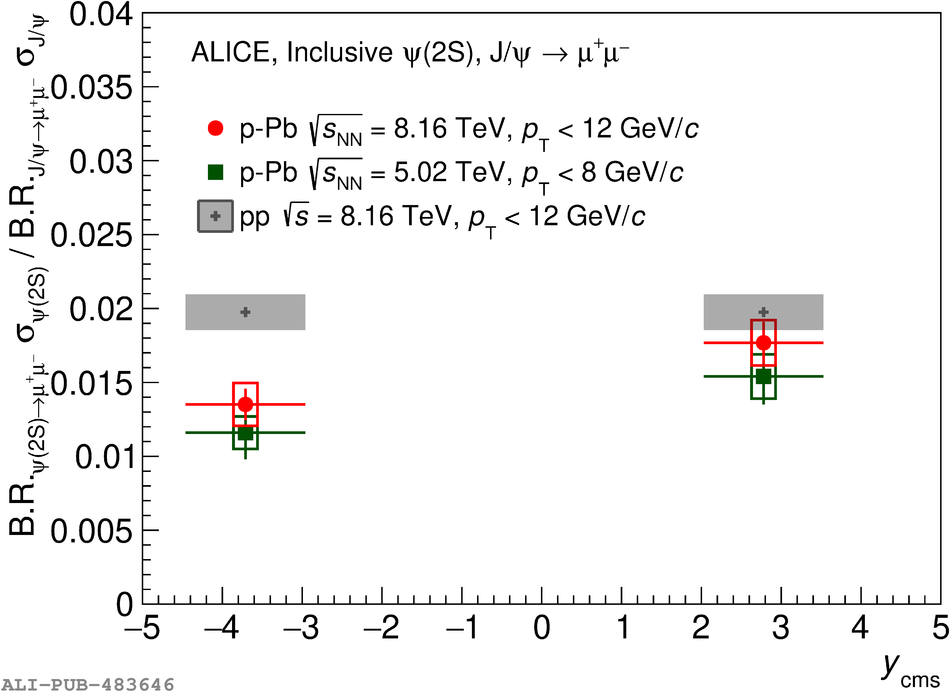# Figure 4

 The ratio ${\rm B.R.}_{\psi(2{\rm S})\rightarrow\mu^+\mu^-}\sigma^{\psi(2{\rm S})}/{\rm B.R.}_{{\rm J}/\psi\rightarrow\mu^+\mu^-}\sigma^{{\rm J}/\psi}$ as a function of $y_{\rm cms}$ for \ppb collisions at $\sqrtSnn = 8.16$ TeV, compared with the corresponding pp quantity, shown as a grey band and obtained via an interpolation of results at $\sqrt{s}=$ 5, 7, 8 and 13 TeV . The error bars represent the statistical uncertainties, while the boxes correspond to uncorrelated systematic uncertainties. The published \ppb results at $\sqrtSnn = 5.02$ TeV  are also shown.